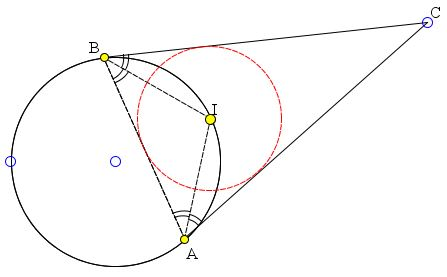# Sangaku with Angle between a Tangent and a Chord

The applet below illustrates a 1896 Sangaku from the Ibaragi prefecture ([Fukagawa & Pedoe], problem 1.1.4, the tablet has vanished.)

The tangents at points A and B on a given circle Γ intersect at C. Show that the incenter I of triangle ABC lies on Γ.

### If you are reading this, your browser is not set to run Java applets. Try IE11 or Safari and declare the site https://www.cut-the-knot.org as trusted in the Java setup.What if applet does not run?

Solution

### References

1. H. Fukagawa, D. Pedoe, Japanese Temple Geometry Problems, The Charles Babbage Research Center, Winnipeg, 1989

Write to:

Charles Babbage Research Center
P.O. Box 272, St. Norbert Postal Station
Winnipeg, MBThe tangents at points A and B on a given circle Γ intersect at C. Show that the incenter I of triangle ABC lies on Γ

Let I' be the midpoint of the small arc AB of Γ.The arcs AI' and BI' are equal. The inscribed angle ABI' equals half the arc AI', angle BAI' equals half the arc BI'. The two angles CAI' and CBI' between the tangents to Γ and the chords AI' and BI' equal respectively half the arcs AI' and BI'. It follows that ∠CAI' = ∠BAI' and ∠CBI' = ∠ABI' (all four are in fact equal.) Thus AI' and BI' are angle bisectors of the angles at A and B of ΔABC, making I' = I, the incenter of the triangle.## Sangaku